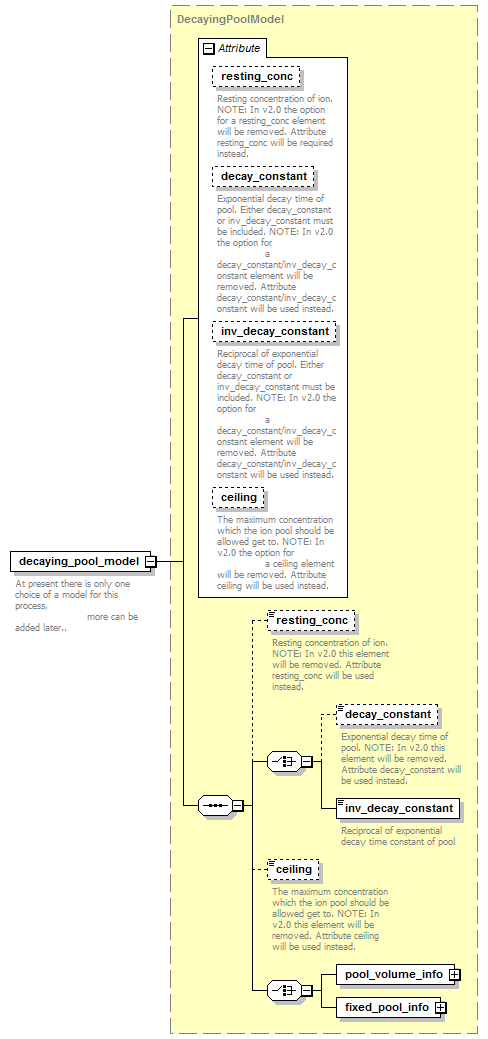# decaying_pool_model

 ```At present there is only one choice of a model for this process, more can be added later..```

## Model## Attributes

QName Type Fixed Default Use Inheritable Annotation
ceiling ConcentrationValue optional
 ```The maximum concentration which the ion pool should be allowed get to. NOTE: In v2.0 the option for a ceiling element will be removed. Attribute ceiling will be used instead.```
decay_constant TimeConstantValue optional
 ```Exponential decay time of pool. Either decay_constant or inv_decay_constant must be included. NOTE: In v2.0 the option for a decay_constant/inv_decay_constant element will be removed. Attribute decay_constant/inv_decay_constant will be used instead.```
inv_decay_constant InvTimeConstantValue optional
 ```Reciprocal of exponential decay time of pool. Either decay_constant or inv_decay_constant must be included. NOTE: In v2.0 the option for a decay_constant/inv_decay_constant element will be removed. Attribute decay_constant/inv_decay_constant will be used instead.```
resting_conc ConcentrationValue optional
 `Resting concentration of ion. NOTE: In v2.0 the option for a resting_conc element will be removed. Attribute resting_conc will be required instead.`

## Source

 ``` At present there is only one choice of a model for this process, more can be added later.. ```